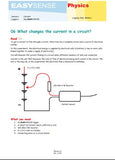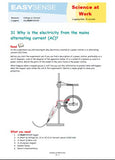# Current - 100mA

datalogging sensors

3166

### +/- 100mA low range, high resolution current sensor for d.c. and low-voltage a.c. measurements. Use in conjunction with voltage sensors to investigate Ohm’s law, power and circuits.

28 -sec Change in magnetic field and induced current

1-minute Data Harvest capacitor - charge & dischargeTeaching applications:
• What changes the current in a circuit?
• Can you use bulb brightness to measure current?
• Ohm’s law
• Series and parallel circuits
• Alternative power investigations e.g. solar cells• Electrical component characteristics e.g. a light dependent resistor
• Capacitor discharge, charge and energy stored
• Faraday’s induction in a coil, induction of current in a conductor
• Power
• Heat and electric current

Sensor Ranges:

Range Name Value Resolution Accuracy
Current 100mA ±100mA 50 µA

### Contents/Details:

±100 mA (Resolution 50 µA)
Maximum Voltage ±27 V
Resistance/Impedance OR8 (1.8 Ohms)
• Offset removed by Tare function in software.
• Be aware that some power supplies are ½ wave rectified producing an average rather than true DC. The Current Sensor will ‘pick up’ the fluctuations in voltage and current from this type of power supply.
• The Current sensor should be placed in series with the circuit component through which the current is measured. Currents in either direction can be measured. The Current sensors have a very low resistance so that they introduce as little resistance as possible to the circuit.Good and bad conductors (Chemistry (11 -14) eBook)
This activity is a standard practical comparing current flow with different materials in a circuit. It is useful for introducing the concepts of dividing elements into groupings determined by the elements physical characteristics, reactions, etc.
Good and bad conductors (Chemistry (14-18) eBook)
This activity is a standard practical comparing current flow with different materials in a circuit. It is useful for introducing the concepts of dividing elements into groupings determined by the elements physical characteristics, reactions, etc.
Faraday's laws of electrolysis (Chemistry (14-18) eBook)
In this investigation the mass lost from a copper electrode is used. Copper is used for convenience, it is readily available in sheet form and can easily be cut into electrodes. Calculate coulombs per faraday.Electricity from water power (Physics (11-14) eBook)
Use a simple water wheel to make electricity via generator. Monitor the voltage and or current over time against other variables e.g. flow, fall height etc.

Current In a circuit adding cells (Physics (11-14) eBook)
How does the current vary with the number of cells added to the battery?

Can lamp brightness be used to measure current? (Physics (11-14) eBook)
This activity involves pupils thinking in detail about the relationship between brightness and current. It makes use of a Current and Light Level sensor to test a qualitative method of measuring current.

What changes the current in a circuit? (Physics (14-18) : Electricity & Heat eBook)
Simple (revision) exercise to see how the number of cells in circuit changes the current,

What changes the current in a circuit (Physics (14-18) : Electricity & Heat eBook)
Alternative exercise using snapshot to collect data instead of reading values off a meters screen.

Good and bad conductors (Physics (14-18) : Electricity & Heat eBook)
This activity is a standard practical comparing current flow with different materials in a circuit. Strips of different conductors and insulators are provided for the pupils. Conductors are anything that let a current flow.

Connecting resistors (Physics (14-18) : Electricity & Heat eBook)
Shows the current in circuit with different resistor values and combinations. Leads to resistor calculations.

Current and brightness In a circuit (Physics (14-18) : Electricity & Heat eBook)
This activity involves pupils thinking in detail about the relationship between brightness and current. Good opportunity to remind about the difference between voltage and current.

Current and voltage (Physics (14-18) : Electricity & Heat eBook)
Introductory activity to explore the relationship between current and voltage with an ohmic conductor. In this variant changes in voltage and current are measured against time.

Current and voltage (Physics (14-18) : Electricity & Heat eBook)
Introductory activity to explore the relationship between current and voltage with an ohmic conductor. In this variant changes in voltage and current are plotted directly against each other, leading into characteristics of conductors / components.

Electrical characteristics (LED, resistor and filament lamp) (Physics (14-18) : Electricity & Heat eBook)
Create voltage current curves for LED, resistor and filament lamp. This worksheet assumes all three curves in one teaching session. 35a and 35b restrict to fewer devices per session.

Electrical characteristics (resistor and filament lamp) (Physics (14-18) : Electricity & Heat eBook)
Specific worksheet to produce voltage current curves for a resistor and filament lamp (ohmic and non ohmic resistance)

Electrical characteristics (Led, diode) (Physics (14-18) : Electricity & Heat eBook)
Restricted instructions for voltage current curves for diodes.

Capacitors: charge stored. (Physics (14-18) : Electricity & Heat eBook)
Collect voltage and current data during a capacitor charge and discharge cycle. Use built in tools to find the energy transferred and stored.

Energy stored on a capacitor (Physics (14-18) : Electricity & Heat eBook)
This investigation allows the student to calculate the energy stored in the capacitor, it is an extension of the work started in 37 Charge stored on a capacitor.

Induction of a current in a conductor (Physics (14-18) : Electricity & Heat eBook)
This activity is a useful demonstration of Faraday's experiments into induction. The current produced is small but the combination of the fast logger and software reveals the induced current easily. Uses a single conductor wire.

Water from electric (Physics (14-18) : Electricity & Heat eBook)
In the experiment the user is asked to investigate the relationship between height of water (the head) and / or the flow of the water over a simple turbine. Opportunity for investigation and design,

How does the magnetic field change along the axis of a coil? (Physics (14-18) : Electricity & Heat eBook)
This investigation will allow the students to understand the way the field changes along the axis of a coil. It uses the EASYSENSE software’s Snapshot facility with a function that asks the user for a value (in this case the distance) after each data collection.

Input characteristic of a transistor (Physics (14-18) : Electricity & Heat eBook)
This investigation is going to study the input characteristics of the transistor across the B–E terminals by applying an alternating voltage, and measuring the voltage across and the current flowing through the B-E junction.

Transistor as a switch and current amplifier (Physics (14-18) : Electricity & Heat eBook)
This investigation will show the students that, 1. The base voltage controls the collector emitter current. 2. A small change in the base voltage causes a large change in the collector voltage. 3. The use of this change in the collector voltage to provide switching. Amplification of current is also considered.

Reactance and phase in a capacitor (Physics (14-18) : Electricity & Heat eBook)
You are going to measure the current through and voltage across the capacitor at different frequencies. From the data, you will calculate the reactance Xc of the capacitor at different frequencies.

Impedance and phase difference with frequency (Physics (14-18) : Electricity & Heat eBook)
Investigate how, in a series C-R circuit, resistance and capacitance combine to affect the flow of an ac current.

L-R circuits: How does Impendance change with frequency? (Physics (14-18) : Electricity & Heat eBook)
The investigation will show: 1. How impedance of a series l-R circuit increases with frequency. 2. The formula for calculating impedance of a series L-R circuit

Resonance In an LCR circuit (Physics (14-18) : Electricity & Heat eBook)
To find the frequency at which the impedance becomes purely resistive - the resonant frequency.

An experimental estimation of Planck's constant (Physics (14-18) : Light, Sound & Pressure eBook)
Uses LEDs of known frequency and measures the forward voltage for first illumination. A plot of turn on voltage vs wavelength leads to an estimate of the constant.Why is electric ac (Science At Work (11-16) eBook)
This experiment tries to show why it is that the electricity supply is created as an alternating current (AC).

HK\$ 550.00

3166